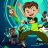# VWAP difference between ToS and TradingSim

Z

##### New member
Hello all,
I use tradingsim and thinkorswim for my strategy backtesting. I use the VWAP indicator for one of my strategies, however the VWAP plot is different on these two platforms.

I think the reason is if the VWAP on thinkonswim uses the HIGH price and VWAP on tradingism uses the Open price for its parameters. I have attached a photo from the two platforms that illustrates this perfectly. Any clarification for the difference?

Any opinions on how to fix this so they are showing the same VWAP?Last edited:
Z

##### New member
I can¨t change the paramters on tradingsim. I guess its possible to do so on TOS with some coding, but unable to find out.

C

#### codydog

##### Member
imo, there's no one best way to calculate vwap, heres an example for tos.

# VWAPALOOZA
#
# This Thinkscript calculates four different types of VWAP:
# OneDay (standard type), Rolling (N bar lookback), Bar
# Incremental (discrete N bar periods) and Volume Incremental
#
declare upper;
#
# Check if we are on the current day:
#
def istoday = if getDay() == getLastDay() then 1 else 0;
#
# Input Rolling / Incremental period, VWAP type, and
# Averaging method ( (H+L)/2 or Close):
#
input Period = 20;
def n = Period;
input VolIncrement=20000;
def v=VolIncrement;
input VWAPType = {Rolling, BarIncremental, VolIncremental, default OneDay};
def type;
switch (VWAPType){
case Rolling:
type = 1;
case BarIncremental:
type = 0;
case OneDay:
type = 2;
case VolIncremental:
type=3;
}
input AverageMethod = {CLOSE, default H_L};
def num;
switch (AverageMethod){
case CLOSE:
num = close;
case H_L:
num = (high + low) / 2;
}
#
# Define volume for today only (other days zero):
#
def todayvol = if istoday then volume else 0;
#
# Calculate the P*V term for VWAP:
#
def pv = num * todayvol;
#
# Code for BarIncremental counter:
#
rec k = if k == n then 1 else k + 1;
#
# Code for VolIncremental volume sum:
#
rec volsum=if volsum>v then volume else volsum+volume;
#
# Code for VolIncremental price*volume:
#
rec pvsum =if volsum>v then num*volume else pvsum + num*volume;
#
# Now define the VolIncremental to display:
#
rec volinc=if volsum==0 then num else if volsum>v then pvsum/volsum else volinc;
#
# Now the final VWAP calculations:
#
rec calcVWAP = if type == 2 then TotalSum(pv) / TotalSum(todayvol) else if type == 1 then sum(num*volume, n) / sum(volume, n) else if type==3 then volinc else if type==0 and k == n then sum(num*volume, n) / sum(volume, n) else calcvwap;
#
# Define the plot:
#
plot VWAPALOOZA = calcvwap;
#
# Formatting:
#
VWAPALOOZA.setdefaultcolor(color.white);
VWAPALOOZA.setstyle(curve.long_dash);
VWAPALOOZA.setlineweight(3);

•BenTenCustom 11